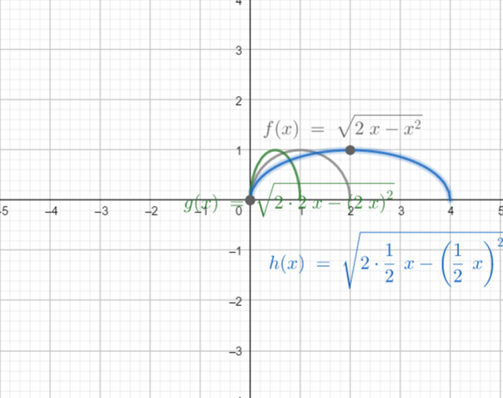# To graph the functionson the same screen and how they related to the graph in part (a). The functions are given below: f ( x ) = 2 x − x 2 ( a ) y 1 = f ( x ) ( b ) y 2 = f ( 2 x ) ( c ) y 3 = f ( 1 2 x )### Precalculus: Mathematics for Calcu...

6th Edition
Stewart + 5 others
Publisher: Cengage Learning
ISBN: 9780840068071### Precalculus: Mathematics for Calcu...

6th Edition
Stewart + 5 others
Publisher: Cengage Learning
ISBN: 9780840068071

#### Solutions

Chapter 2.5, Problem 73E
To determine

## To graph the functionson the same screen and how they related to the graph in part (a).The functions are given below:  f(x)=2x−x2(a) y1=f(x)(b)y2=f(2x)(c)y3=f(12x)

Expert Solution

### Explanation of Solution

Given information:

The function are:

f(x)=2xx2(a) y1=f(x)(b)y2=f(2x)(c)y3=f(12x)

Graph :

the graph obtained as:Interpretation: from the above graph it is observed that for part(b) shrink graph of part (a) horizontally by a factor of 12 .

For part (c) stretchthe graph of part (a) horizontally by a factor of 2.

### Have a homework question?

Subscribe to bartleby learn! Ask subject matter experts 30 homework questions each month. Plus, you’ll have access to millions of step-by-step textbook answers!